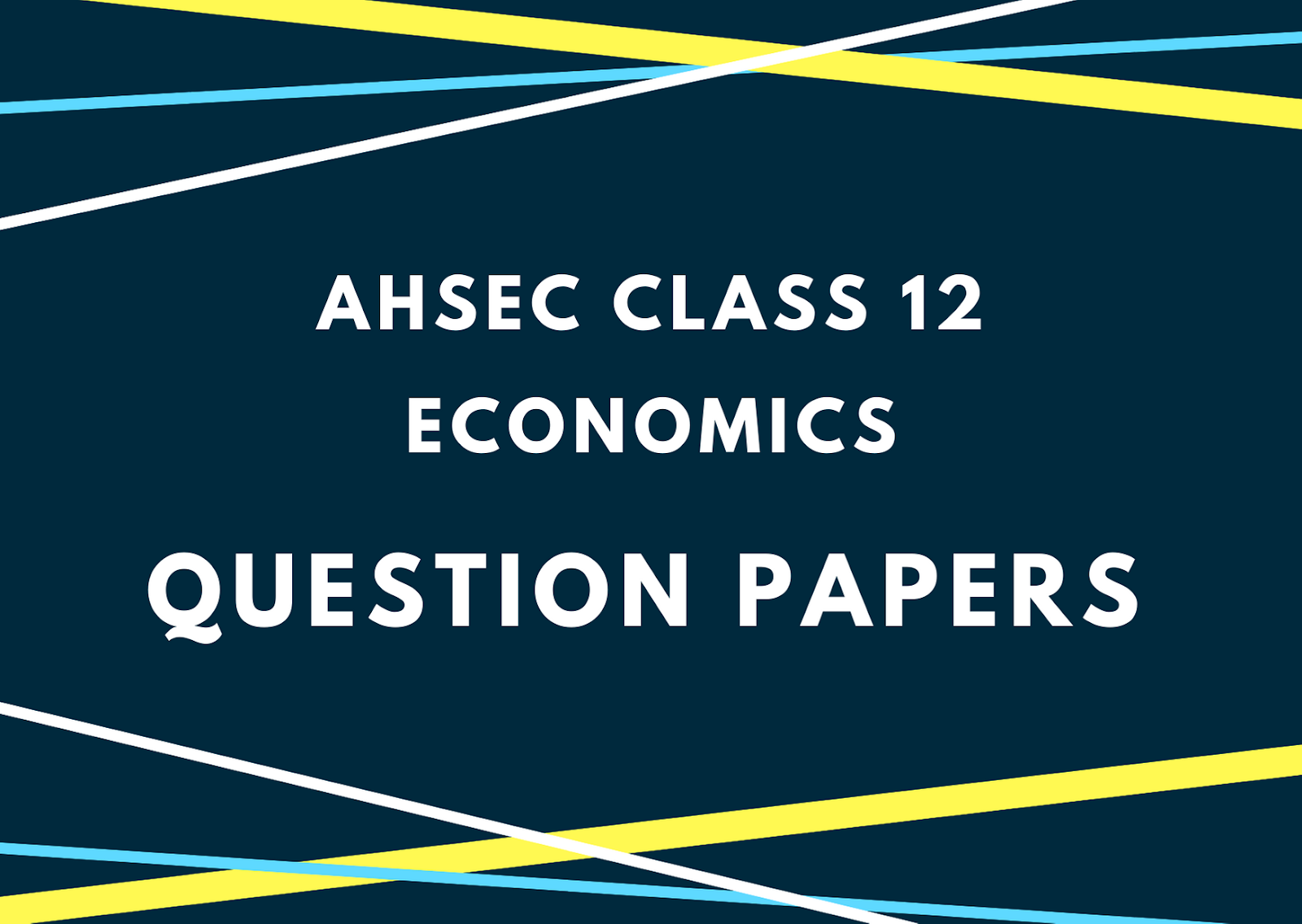# AHSEC Class 12 Economics Question Paper 2015## AHSEC Class 12 Economics Question Papers 2015ACCOUNTANCYFull Marks: 100Pass Marks: 30Time: Three HoursFull Marks: 100 Time: 3 hour
1. (a) Fill in the blanks:
In economics, it is generally assumed that the consumer is a ____ individual. 1
(b) Define substitute goods. 1
(c) Define inferior goods. 1
(d) In which form of market, products are homogeneous? 1
(e) What is the shape of supply curve in the market period? 1
(f) What is meant by equilibrium price? 1
2. Give the concept of centrally planned economy. 2
3. Distinguish between positive economics and normative economics. 2
4. Draw a vertical demand curve and state the nature of price elasticity on it. 2
5. State any two assumptions of the law of demand. 2
6. What is shut down point of a firm? 2

7. Total fixed cost of a firm is Rs. 100 when it produces 15 units of output. If the level of output increases to 30 units, what will be the fixed cost in the short-run? Given reasons for your answer. 2
8. Distinguish between change in quantity supplied and change in supply. 4
9. Write down four characteristics of perfectly competitive market. 4
10. Explain with the help of a diagram how the shifting of the demand curve for a commodity affects the equilibrium price and output. 4
Or
What is change in demand? State any three factors that can cause shift in the demand curve.
11. State the law of diminishing returns. State the reason behind this law. 1+3=4
Or
Explain the relationship between Marginal Product (MP) and Total Product (TP) of an input.
12. Distinguish between explicit cost and implicit cost. Give one example of each of them. 2+2=4
13. Explain with the help of a diagram why at consumer’s optimum point the budget line should be tangent to an indifference curve. 6
Or
Explain the conditions for profit maximization of a firm.
14. Show with the help of a diagram how the market demand curve can be derived from individual demand curves. 6
Or
Explain the relation among TR, AR and MR of a monopolist with the help of a hypothetical table and a diagram.15. (a) What is macroeconomics? 1
(b) What is circular flow of income? 1
(c) Define intermediate goods. 1
(d) What is velocity of circulation of money? 1
(e) “The speculative demand for money is ……………. (directly/inversely) related to the market rate of interest.”(Fill in the blank by choosing the correct word from the bracket.) 1
(f) What is foreign exchange rate? 1
16. Explain the concept of depreciation in the context of national income accounting. 2
17. If the marginal propensity to consumer (C) of an economy is 0.9, find out the value of the income multiplier.    2

18. Distinguish between consumption goods and capital goods? 2
19. Explain the significance of revenue deficit. 2
20. Define private goods and public goods. 2
21. State two merits of fixed exchange rate. 2
22. What do you understand by the problem of double counting? Explain the need for avoiding double counting in the estimation of national income. 2+2=4
23. It is planned to make a new investment of Rs. 1000 crores in a economy. How much will be the increase in National Income if MPS is 0.4? 4
24. What is investment? Distinguish between gross investment and net investment. 2+2=4
25. Explain two functions operated through government revenue and expenditure measures. 4
26. Explain the concepts of autonomous and accommodating transactions of balance of payments. 4
Or
What do you mean by disequilibrium in balance of payment (BOP)? Mention any two causes of adverse BOP of a country.

27. From the following data, find out personal income and personal disposable income. 4+2=6
 Rs. in crore NDP at factor cost Net factor income from abroad Undistributed profit Corporate Tax Interest received by household Interest paid by household Transfer income Personal tax 9,000 150 500 600 1,200 1,000 400 600

Or
Explain the income method of calculating GDP.
28. Briefly explain any four functions of a commercial bank. 6
Or
Explain three instruments of credit control used by the central bank.